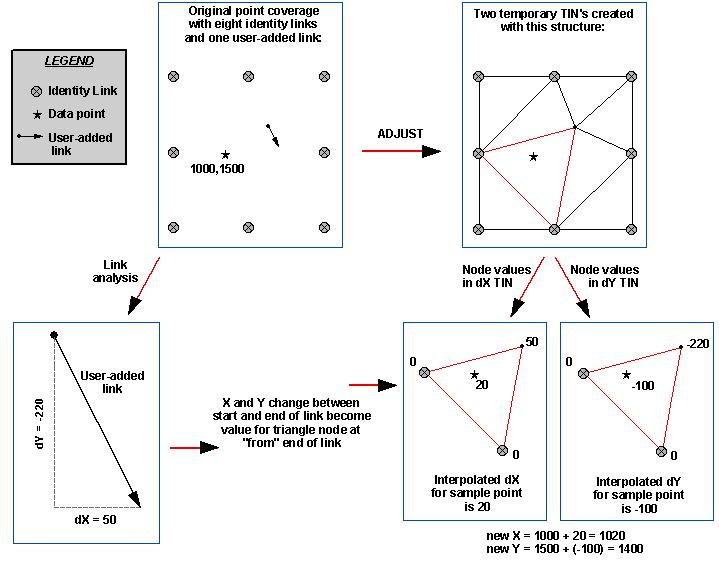HOW TO

# Understand TIN interpolation process used by ADJUST

Last Published: April 25, 2020

## Summary

A description of how the ADJUST command uses triangulation to determine how to move input feature coordinates relative to user-specified links.

## Procedure

ADJUST uses two temporary triangulated irregular networks (TINs) to interpolate changes in x (dX) and changes in y (dY) for feature coordinates along user-specified links. Each TIN has the same triangulation structure. The from-end of the links and all identity links are used as the TIN triangle corners (nodes). A node is defined by its x,y location and a z value.

The z value of each node is used to interpolate the amount of x,y adjustment applied to each feature coordinate in the coverage. The z value is the amount of change between the from-end and to-end of a link. For example, if the change in x for a link is 10 map units, the z value of the TIN node at the from-end of that link will be 10. Since identity links represent no change, the z value is zero. Once each node of a TIN triangle has a z value, the corresponding z value of any point falling on that triangle can be interpolated.

The interpolated z value from the x-shift TIN is added to the x ordinal of the feature's coordinate. The z value interpolated from the y-shift TIN is added to the y ordinal of the coordinate. For example, if an input feature coordinate were 1000,1500, the interpolated dX for this point is 20, and the interpolated dY is -100, the output coordinates after ADJUST will be 1020,1400 (1000 + 20 = 1020 and 1500 + (-100) = 1400).`Note:General information about the ADJUST process can be found in the online ArcDoc section:Contents > Data automation > Editing coverages and INFO file with ARCEDIT > Rubber sheeting > What happens during ADJUSTGeneral information regarding TIN structure can be found in the ArcDocsection:Contents > Spatial Modeling with TINGeneral information about linear and bivariate quintic interpolation can befound in the ArcDoc section:Contents > Spatial Modeling with TIN > Surface concepts > TIN interpolatorsFor specifics for the mathematical models of the interpolators, see:Contents > Spatial Modeling with TIN > Suggestions for further reading in TIN > Akima, H., “A Method of Bivariate Interpolation and Smooth Surface Fitting for Irregularly Distributed Data Points.” ACM Transactions on Mathematical Software 4 (June 1978): 148-159.`

Article ID:000002520

Software:
• Legacy Products
• ArcMap 8 x

Get help from ArcGIS experts

Contact technical support